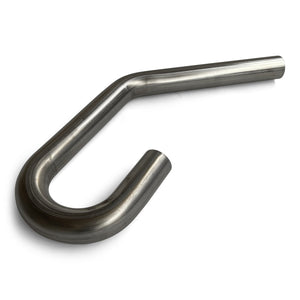# 304 Stainless Steel UJ Combo Bend

• \$19.99
Unit price per

This combo bend has a 45 degree bend and a U-bend, which can be cut down into two 90 degree bends. Great for building down pipes and plumbing waste gates back into the exhaust.

Refer to the image and the table below for exact measurements:
1.5" - A = 5", B = 8", C= 8", R=  2.25"
1.75" - A =  5", B = 7", C= 7", R= 2.63"
2.0" - A = 4", B = 9", C= 12", R= 3"
2.5" - A = 5", B = 8", C= 12", R= 3.75"
3.0" - A = 8", B = 10", C= 23", R= 4.5"
3.5" - A = 7", B = 13", C= 21", R= 5.25"
4.0" - A = 7", B = 15.75", C= 32", R= 6"